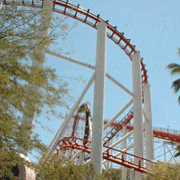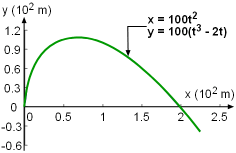Ch 10. Parametric Equations Multimedia Engineering Math ParametricEquations Tangentsand Areas Arc Length Surface Area
 Chapter 1. Limits 2. Derivatives I 3. Derivatives II 4. Mean Value 5. Curve Sketching 6. Integrals 7. Inverse Functions 8. Integration Tech. 9. Integrate App. 10. Parametric Eqs. 11. Polar Coord. 12. Series Appendix Basic Math Units Search eBooks Dynamics Fluids Math Mechanics Statics Thermodynamics Author(s): Hengzhong Wen Chean Chin Ngo Meirong Huang Kurt Gramoll ©Kurt GramollMATHEMATICS - CASE STUDY IntroductionRoller Coaster During a field trip to an amusement park, a teacher asked her students to determine the slope at a specific point of the roller coaster ride. A portion of the path of the ride can be described using the following parametric equations:      x = 100t2      y = 100(t3 - 2t) for -1.5 ≤ t ≤ 0. QuestionsPath Described by Parametric Equations x = 100t2 and y = 100(t3 - 2t) Determine the tangent when t is -1.1. Approach Note that the parametric equations are defined for an interval of t only (i.e., -1.5 ≤ t ≤ 0).